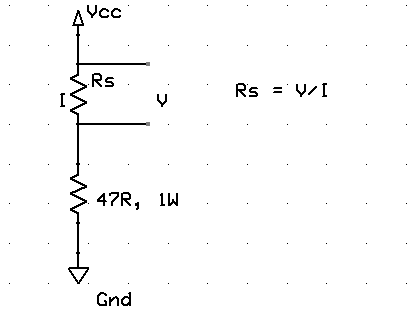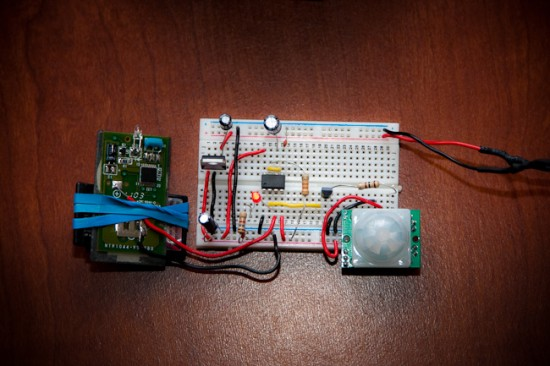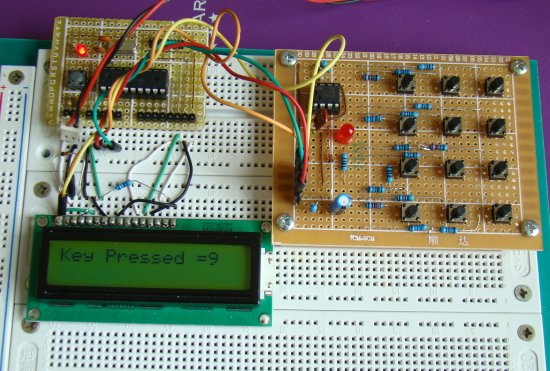## How to measure dc current with a microcontroller?Microcontrollers usually don’t have specific ports for measuring currents, but they do have ADC channels through which you can measure analog voltages of a certain range. This means a dc current can be indirectly measured by a microcontroller’s ADC channel by first converting the current into voltage. The simplest way of doing this is to place a resistance in series with the current path and measure the voltage drop across it. But hold on, if you place an additional resistance in the circuit, it will affect the original current. Therefore, we need to use a very small value resistance so that it’s effect in the circuit current won’t be significant.

Resistors with values less than 1 ? are available in electronics stores. Depending upon the amount of current in the circuit, you need to choose proper power rating for the resistor. Suppose, if you pick 0.47 ?, and the maximum current in the circuit is about 2 A, then the resistor should have the capacity of dissipating 4 x 0.47 ? 2 Watts of heat.

You can also make a small value resistance by yourself. Yes, by simply winding a copper wire into coil. I have made one from a 5 ft long solid copper wire (22 AWG) with plastic insulation on outer side, as shown below.

Now lets measure its resistance. The resistance can be measured directly with a digital multimeter. My digital meter shows its value equal to 0.3 ?. This measurement may have higher uncertainty as it is very small and most multimeter does not show values beyond 1 decimal digit. The resistance can also be measured using Ohm’s law. Connect a 47? ? resistor in series with the coil resistance (Rs) and supply a 5V power as shown below. Next, measure the voltage across Rs and current through it separately using the multimeter. In my case, I found the measured voltage and current values to be 24.1 mV and 84.3 mA, respectively. This gives the resistance of the coil is about 0.286 ?.Now, suppose that the range of current to be measured using this coil resistance is from 0-2 A. Then the voltage drop across the coil resistance will be somewhere from 0 – 0.57 V. Because of its low dynamic range, this voltage signal may not be accurately measured with a microcontroller’s ADC module. So this requires some sort of voltage scaling. One way to achieve that is by using an operational amplifier circuit as shown below.

In the circuit, Rs is the low value current sensing resistor (our coil resistor) which is connected in series with the load resistor. Our objective is to derive the load current (I). The low voltage drop across Rs is amplified by the non-inverting amplifier. The gain of the amplifier is set by Rf and Ri resistors. For Rf = 10 K, and Ri = 1.3 K, the gain of the amplifier would be about 8.7. This is enough to linearly scale Vs (0-0.57 V) to Vo (0- ?5 V). Now you have 0-5 V voltage signal that corresponds to 0-2 A current through Rs. This voltage signal is now more appropriate for ADC conversion with Vref = 5 V.

Vo = 8.7 x I x Rs = 2.49I (Rs = 0.286 ?)

=> I = Vo/2.49.

For 10-bit ADC with Vref = 5 V, resolution = 5/1024 = 0.0049 V. For input signal Vo, the ADC O/P will be Vo x 0.0049. Thus,

The current resolution would be therefore 0.00197 A (? 2 mA).

## 555 Contest Entry: Motion activated cameraThis entry for the 555 timer contest is from Andrew Smith who built a motion activated switch for a digital camera. The 555 timer is operating in monostable mode which is triggered by a PIR sensor when motion is detected. The monostable output of 555 then activates the camera through a remote.## 555 Contest Entry: Automatic hair drierHere’s another entry for the 555 contest. This project is about an automatic hand dryer that turns on when the hands are brought close to the dryer. It uses infrared diodes and a photo diode sensor to detect the presence of hands. The optical sensor mechanism then triggers the 555 timer which is configured in a monostable mode. The 555 output activates a relay switch that turns the fan on. The duration of the monostable output (fan on time) is defined by the resistance and capacitance connected to 555. Read more

## 555 Contest Entry: AM radio555 timer based AM radio receiver published on Tube Time is one of many entries for the currently running 555 contest. This project uses a 555 timer as AM demodulator plus amplifier to drive the speaker. The radio signal is tuned with an LC tank circuit. The 555 timer is configured as a PWM where a ramp signal is created with a capacitor and a potentiometer. The radio signal picked by the LC circuit is superimposed on the ramp signal which varies the duty cycle of the output PWM wave. The variation in the duty cycle corresponds to the audio signal in the radio waves. Read more

## 2-Wire Keypad Interface Using a 555 TimerKeypads are a very commonly used input device in microcontroller-based systems. In a keypad, multiple switches are arranged in rows and columns so that they could be interfaced to a microcontroller with a minimum number of I/O pins. For example, a 12-key keypad is arranged in a 4×3 format, which allows to interface the 12 keys to a microcontroller with only 7 connections. The location of each key on the keypad is defined by two coordinates: the row and the column. When a key is pressed, it connects its row with its column. The microcontroller must scan all the rows and columns to find out which key has been pressed. This is the most common way of interfacing a keypad to a microcontroller. There are tons of resources on the internet regarding this technique and so I am not going to discuss it here.

Today, I am going to share with you about a new keypad interfacing technique that uses only two I/O pins of a microcontroller: one for signalling the microcontroller when a key is pressed, and the other is to read the key information. It is based on a 555 timer IC which is configured as an astable multivibrator. I am not sure if anybody has ever tried using a 555 IC for keypad interfacing, but this technique really works. I am going to demonstrate this with a 4×3 keypad (a standard telephone dial pad). A PIC16F628A microcontroller will read the output of a 555 timer IC, determine what key has been pressed, and display it on a character LCD module.